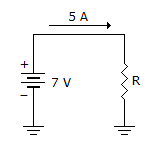# Electronics - Ohm's Law

## Why Electronics Ohm's Law?

In this section you can learn and practice Electronics Questions based on "Ohm's Law" and improve your skills in order to face the interview, competitive examination and various entrance test (CAT, GATE, GRE, MAT, Bank Exam, Railway Exam etc.) with full confidence.

## Where can I get Electronics Ohm's Law questions and answers with explanation?

IndiaBIX provides you lots of fully solved Electronics (Ohm's Law) questions and answers with Explanation. Solved examples with detailed answer description, explanation are given and it would be easy to understand. All students, freshers can download Electronics Ohm's Law quiz questions with answers as PDF files and eBooks.

## Where can I get Electronics Ohm's Law Interview Questions and Answers (objective type, multiple choice)?

Here you can find objective type Electronics Ohm's Law questions and answers for interview and entrance examination. Multiple choice and true or false type questions are also provided.

## How to solve Electronics Ohm's Law problems?

You can easily solve all kind of Electronics questions based on Ohm's Law by practicing the objective type exercises given below, also get shortcut methods to solve Electronics Ohm's Law problems.

### Exercise :: Ohm's Law - General Questions

1.

"Half-splitting" is

 A. a means for nuclear fuel cells to produce electricity B. a means of isolating a problem in a circuit C. a means for reducing a high ac voltage to a low dc voltage D. a means of limiting current in a circuit

Explanation:

No answer description available for this question. Let us discuss.

2.

If doubling the voltage across a resistor doubles the current through the resistor then

 A. the resistor value decreased B. the resistor value did not change C. the resistor value increased D. it is impossible to determine the change in the resistor value

Explanation:

No answer description available for this question. Let us discuss.

3.

If the voltage across a fixed value of resistance is increased five times, what does the current do?

 A. It increases by a factor of five. B. It decreases by a factor of five. C. It stays the same. D. Not enough information

Explanation:

No answer description available for this question. Let us discuss.

4.

If the resistance in a circuit with constant voltage increases, the current will

 A. increase B. decrease C. stay the same D. Not enough information

Explanation:

No answer description available for this question. Let us discuss.

5.What is the power in the given circuit?

 A. 3.6 W B. 35 W C. 175 W D. 245 W